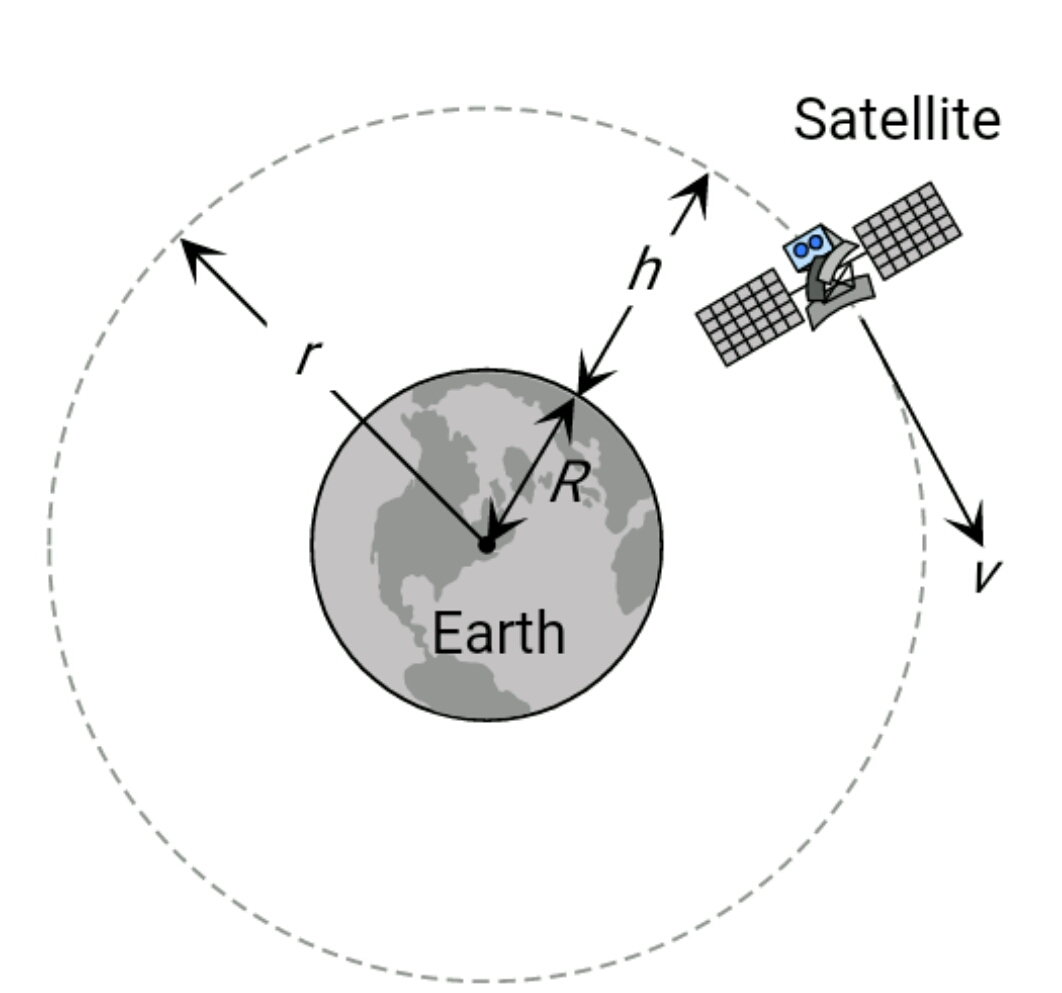#### If suddenly the gravitational force of attraction  between Earth and a satellite revolving around it becomes zero, then the satellite will : Option 1) continue to move in its orbit with same velocity Option 2) move tangentially to the original orbit in the same velocity Option 3) become stationary in its orbit Option 4) move towards the earthAs we learnt in

Orbital velocity of satellite -Position of satellite from the centre of earthOrbital velocity

- wherein

The velocity required to put the satellite into its orbit around the earth.

The centripetal and centrifugal forces disappear, the satellite has the tangential velocity and it will move in a straight line.

Option 1)

continue to move in its orbit with same velocity

This option is incorrect.

Option 2)

move tangentially to the original orbit in the same velocity

This option is correct.

Option 3)

become stationary in its orbit

This option is incorrect.

Option 4)

move towards the earth

This option is incorrect.

##### Posted by